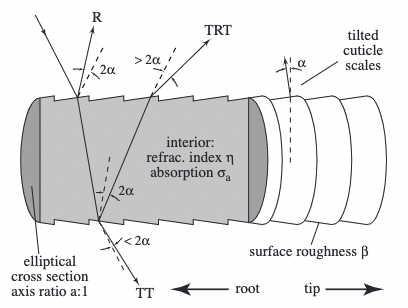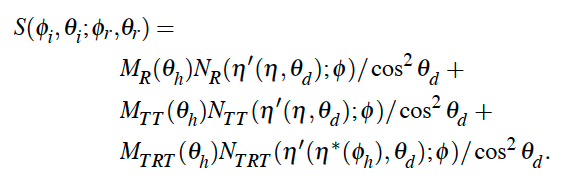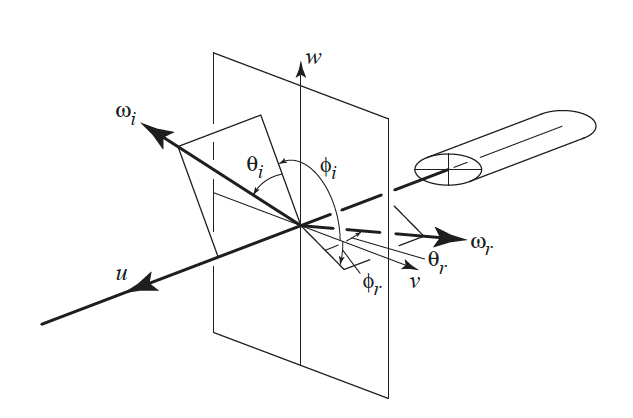# PBR论文实现：Marschner's/d'Eon Hair Model$S(\phi_i, \theta_i;\phi_r,\theta_r) = M(\theta_i, \theta_r)N(\eta'(\theta_d);\phi_i,\phi_r)/\cos^2(\theta_d)$### Longitudinal散射方程

$M_p(\theta_h)=g(\beta_p; \theta_h - \alpha_p)$### Azimuthal散射方程Marschner模型中对于$$N_p$$项使用了一个带微分的求和公式来准确计算散射波瓣的能量：

$N_p(p, \phi) = \sum_rA(p, h(p,r,\phi))|2\frac{d\phi}{dh}(p, h(p,r,\phi))|^{-1}$

$\phi(p, h) = 2p\gamma_t-2\gamma_i+p\pi$

$A(p,h)=(1-F(\eta',\eta'',\gamma_i))^2F(\frac{1}{\eta'}, \frac{1}{\eta''}, \gamma_t)^{p-1}T(\sigma'_a,h)^p$

$N_p(\phi) = \frac{1}{2}\int^1_{-1}A(p,h)D_p(\phi - \Phi(p,h))dh$

$D_p(\phi) = \sum^{\infty}_{k=-\infty}g(\beta_p;\phi-2\pi k)$## 附录：Mitsuba的编译和配置

mitsuba的官方仓库中是这么介绍自己的：

Mitsuba is a research-oriented rendering system in the style of PBRT, from which it derives much inspiration. It is written in portable C++, implements unbiased as well as biased techniques, and contains heavy optimizations targeted towards current CPU architectures. Mitsuba is extremely modular: it consists of a small set of core libraries and over 100 different plugins that implement functionality ranging from materials and light sources to complete rendering algorithms. In comparison to other open source renderers, Mitsuba places a strong emphasis on experimental rendering techniques, such as path-based formulations of Metropolis Light Transport and volumetric modeling approaches. Thus, it may be of genuine interest to those who would like to experiment with such techniques that haven't yet found their way into mainstream renderers, and it also provides a solid foundation for research in this domain. The renderer currently runs on Linux, MacOS X and Microsoft Windows and makes use of SSE2 optimizations on x86 and x86_64 platforms. So far, its main use has been as a testbed for algorithm development in computer graphics, but there are many other interesting applications. Mitsuba comes with a command-line interface as well as a graphical frontend to interactively explore scenes. While navigating, a rough preview is shown that becomes increasingly accurate as soon as all movements are stopped. Once a viewpoint has been chosen, a wide range of rendering techniques can be used to generate images, and their parameters can be tuned from within the program.

1. 首先从Github上拉取源代码：
1. 重点：切换到支持python3和scons3的分支上
1. 安装对应依赖
1. 提取config
1. 在根目录下使用scons进行编译

1. 设置环境变量

Carbene Hu

2021-12-26

2023-01-08